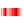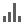Next Previous Up Contents
Next: Gaussian Form
Up: Plot Forms
Previous: KNN Form

#### A.4.5.24 Densogram Form

The Densogram form () represents smoothed density of data values along the horizontal axis using a colourmap. This is like a Kernel Density Estimate (smoothed histogram with bins 1 pixel wide), but instead of representing the data extent vertically as bars or a line, values are represented by a fixed-size pixel-width column of a colour from a colour map. A smoothing kernel, whose width and shape may be varied, is applied to each data point.

This is a rather unconventional way to represent density data, and this plotting mode is probably not very useful. But hey, nobody's forcing you to use it.

This form may be used in either the Histogram, Plane or Time plot windows.Example Densogram plotDensogram form configuration panel

These options always appear in the form configuration panel:

Colour
The base colour for the data set. Only certain shader colour maps respect this value, for others it is ignored.
Combine
If you have a non-blank Weight value, this option controls how the weight values present at each X-axis pixel are combined to give the densogram colour. The default is sum, which makes sense for a weighted or unweighted plot, but other options available are mean, median, min, max and stdev.
The colour map for displaying density values. There are two types, relative and absolute. Relative maps have names marked by a star ("*"), and alter the basic dataset colour, for instance by darkening or lightening it, while absolute maps (the rest) ignore the basic dataset colour altogether. For a single-dataset plot, the absolute maps are best, but for multiple subsets it may be less confusing to use a relative one.
Select a sub-range of the full colour map above. If the Default checkbox is checked, then all or most of the colour ramp from the Shader control is used. If you want to configure the range of colours from the map yourself, uncheck the Default checkbox, and slide the handles in from the end of the slider to choose exactly the range you want.

The default range is clipped at one end for colour maps that fade to white, so that all the plotted colours will be distinguisable against a white background. If you don't want that, you can uncheck Default and leave the handles at the extreme ends of the slider.

Whether the density scale should map forwards or backwards into the colour map.
Allows the colour map to be quantised. By default, the colour map is effectively continuous. If you slide the slider to the right, or enter a value in the text field, the map will be split into a decreasing number of discrete colours. This can be used to generate a contour-like effect, and may make it easier to trace the boundaries of regions of interest by eye.
Scaling
Determines the function used to map the range of density values onto the colour map. Options are linear, logarithmic, square and square root, arc cosine, cosine.
Density Subrange
Adjusts the density range over which the colour map is applied. By default the colour map is scaled using limits found from the data density in the plot (the most dense few pixels are ignored), but you can restrict the range using this slider.
Size
Height of the density bar in pixels.
Position
Determines where on the plot region the density bar appears. The value should be in the range 0..1; zero corresponds to the bottom of the plot and one to the top.
And these options appear in the form configuration panel for the Plane window, or the Bins control () for the Histogram window:
Smoothing
Configures the smoothing width for kernel density estimation. This is the characteristic width of the kernel function to be convolved with the density to produce the visible plot.

Sliding the slider to the right makes the kernel width larger. The width in data units is shown in the text field on the right (if the X axis is logarithmic, this is a factor). Alternatively you can click the radio button near the text field, and enter the width in data units directly.

Kernel
The functional form of the smoothing kernel. The functions listed refer to the unscaled shape; all kernels are normalised to give a total area of unity.

The available options are:

• square: Uniform value: f(x)=1, |x|=0..1
• linear: Triangle: f(x)=1-|x|, |x|=0..1
• epanechnikov: Parabola: f(x)=1-x*x, |x|=0..1
• cos: Cosine: f(x)=cos(x*pi/2), |x|=0..1
• cos2: Cosine squared: f(x)=cos^2(x*pi/2), |x|=0..1
• gauss3: Gaussian truncated at 3.0 sigma: f(x)=exp(-x*x/2), |x|=0..3
• gauss6: Gaussian truncated at 6.0 sigma: f(x)=exp(-x*x/2), |x|=0..6

Cumulative
If set to forward or reverse, the levels are calculated cumulatively; each bin includes the counts from all previous bins in the direction of negative or positive infinity.

Next Previous Up Contents
Next: Gaussian Form
Up: Plot Forms
Previous: KNN Form

TOPCAT - Tool for OPerations on Catalogues And Tables